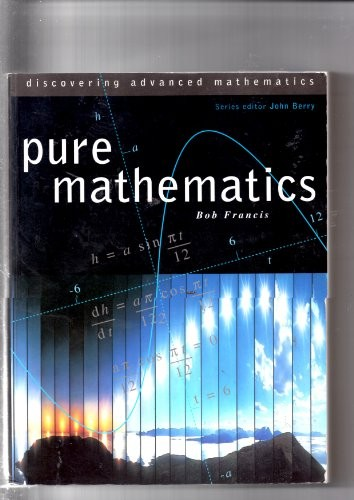# Pure Mathematics by Edited by Bob Francis

In Stock
£4.49
This text shows the immediate application of pure mathematics and makes great use of modelling techniques. The book is divided into 29 short chapters and provides chapter introductions, chapter summaries, investigations and practicals, exercises and examination questions.
Only 1 left

## Pure Mathematics Summary

### Pure Mathematics by Edited by Bob Francis

"Discovering Advanced Mathematics" is a series of four books designed to match the new generation of A and AS Level maths syllabuses and satisfy the requirements of the SCAA Common Core for Mathematics. This text shows the immediate application of pure mathematics and makes great use of modelling techniques. The book is divided into 29 short chapters, allowing teachers to match the book easily to their own teaching plans. It provides chapter introductions, chapter summaries, investigations and practicals, exercises and examination questions.

### Why buy from World of BooksOur excellent value books literally don't cost the earthFree delivery in the UKEvery used book bought is one saved from landfill

Problem-solving in mathematics; basic algebra; linear functions; quadratic functions; quadratic equations and inequalities; polynomial functions; differentiation 1 (gradients etc.); trigonometry 1 (functions etc.); integration 1 (areas, area functions); trigonometry 2 (triangles); sequences and series 1 (notation etc.); indices; functions; sequences and series 2 (binomial expansions); trigonometry 3 (radians etc.); logarithms; exponentials and logarithms; differentiation 2 (negative exponents, tangents); integration 2 (negative exponents etc.); differentiation 3 (chain rule etc.); numerical methods; rational functions; integration 3; curves 1 (parametric equations); trigononmetry 5 (calculus); curves 2; differential equations; mathematics of uncertainty.

GOR001399256
Pure Mathematics by Edited by Bob Francis
Edited by Bob Francis
Used - Good
Paperback
HarperCollins Publishers
1996-06-26
704
0003223701
9780003223705
N/A
Book picture is for illustrative purposes only, actual binding, cover or edition may vary.
This is a used book - there is no escaping the fact it has been read by someone else and it will show signs of wear and previous use. Overall we expect it to be in very good condition, but if you are not entirely satisfied please get in touch with us.